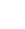Developer

Mac Automation Scripting Guide## Converting RGB to HTML Color

In HTML documents, colors are typically represented as hex values. The handlers in Listing 31-1 and Listing 31-2 show how to convert 8-bit or 256 color-based RGB values to hex values. Provide an RGB color represented by a list of three numbers, each with a value between `0` and `65535`.

APPLESCRIPT

Listing 31-1AppleScript: Handler that converts an RGB color to a hex value
1. `on convertRGBColorToHexValue(theRGBValues)`
2. ` set theHexList to {"0", "1", "2", "3", "4", "5", "6", "7", "8", "9", "A", "B", "C", "D", "E", "F"}`
3. ` set theHexValue to ""`
4. ` repeat with a from 1 to count of theRGBValues`
5. ` set theCurrentRGBValue to (item a of theRGBValues) div 256`
6. ` if theCurrentRGBValue is 256 then set theCurrentRGBValue to 255`
7. ` set theFirstItem to item ((theCurrentRGBValue div 16) + 1) of theHexList`
8. ` set theSecondItem to item (((theCurrentRGBValue / 16 mod 1) * 16) + 1) of theHexList`
9. ` set theHexValue to (theHexValue & theFirstItem & theSecondItem) as string`
10. ` end repeat`
11. ` return ("#" & theHexValue) as string`
12. `end convertRGBColorToHexValue`

JAVASCRIPT

Listing 31-2JavaScript: Function that converts an RGB color to a hex value
1. `function convertRGBColorToHexValue(rgbValues) {`
2. ` var r = parseInt(rgbValues, 10).toString(16).slice(-2)`
3. ` if (r.length == 1)`
4. ` r = "0" + r`
5. ` var g = parseInt(rgbValues, 10).toString(16).slice(-2)`
6. ` if (g.length == 1)`
7. ` g = "0" + g`
8. ` var b = parseInt(rgbValues, 10).toString(16).slice(-2)`
9. ` if (b.length == 1)`
10. ` b = "0" + b`
11. ` return ("#" + r + g + b)`
12. `}`

Listing 31-3 shows how to call the handlers in Listing 31-1 to convert a specified RGB color to a hex value for use in HTML.

APPLESCRIPT

Listing 31-3AppleScript: Calling a handler to convert an RGB color to a hex value
1. `set theRGBValues to (choose color default color {65535, 0, 0})`
2. `convertRGBColorToHexValue(theRGBValues)`
3. `--> Result: "#FF0000"`

JAVASCRIPT

Listing 31-4JavaScript: Calling a function to convert an RGB color to a hex value
1. `var app = Application.currentApplication()`
2. `app.includeStandardAdditions = true`
3. ` `
4. `var color = app.chooseColor({defaultColor: [1, 0, 0]})`
5. `color = [Math.trunc(color * 65535), Math.trunc(color * 65535), Math.trunc(color * 65535)]`
6. `convertRGBColorToHexValue(color)`
7. `// Result: "#FF0000"`